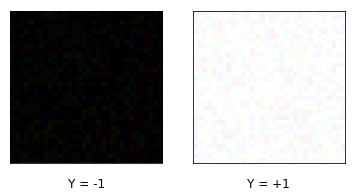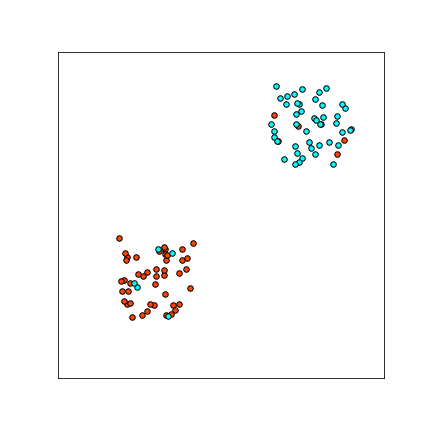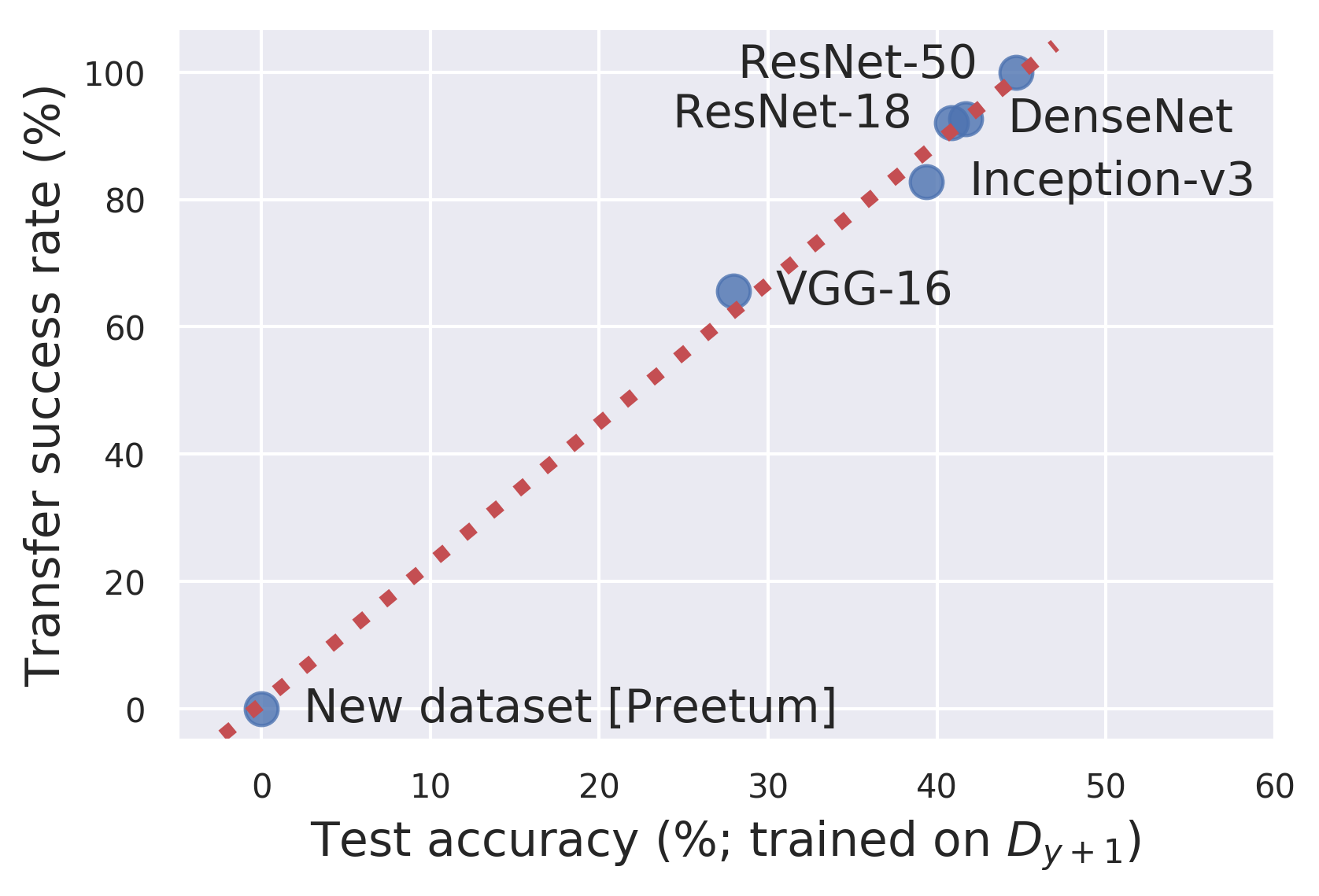$\def\R{{\mathbb{R}}} \def\cY{{\mathcal{Y}}} \def\cX{{\mathcal{X}}} \def\cD{{\mathcal{D}}} \def\eps{\varepsilon} \newcommand{\E}{\mathop{\mathbb{E}}}$

# Adversarial Examples are Just Bugs, Too

Refining the source of adversarial examples

Harvard, OpenAI

Aug. 6, 2019

### DOI

10.23915/distill.00019.5

This article is part of a discussion of the Ilyas et al. paper “Adversarial examples are not bugs, they are features”. You can learn more in the main discussion article .

All Responses Comment by Ilyas et al.

We demonstrate that there exist adversarial examples which are just “bugs”: aberrations in the classifier that are not intrinsic properties of the data distribution. In particular, we give a new method for constructing adversarial examples which:

1. Do not transfer between models, and
2. Do not leak “non-robust features” which allow for learning, in the sense of Ilyas-Santurkar-Tsipras-Engstrom-Tran-Madry .

We replicate the Ilyas et al. experiment of training on mislabeled adversarially-perturbed images (Section 3.2 of ), and show that it fails for our construction of adversarial perturbations.

The message is, whether adversarial examples are features or bugs depends on how you find them — standard PGD finds features, but bugs are abundant as well.

We also give a toy example of a data distribution which has no “non-robust features” (under any reasonable definition of feature), but for which standard training yields a highly non-robust classifier. This demonstrates, again, that adversarial examples can occur even if the data distribution does not intrinsically have any vulnerable directions.

### Background

Many have understood Ilyas et al. to claim that adversarial examples are not “bugs”, but are “features”. Specifically, Ilyas et al. postulate the following two worlds: As communicated to us by the original authors.

• World 1: Adversarial examples exploit directions irrelevant for classification (“bugs”). In this world, adversarial examples occur because classifiers behave poorly off-distribution, when they are evaluated on inputs that are not natural images. Here, adversarial examples would occur in arbitrary directions, having nothing to do with the true data distribution.
• World 2: Adversarial examples exploit useful directions for classification (“features”). In this world, adversarial examples occur in directions that are still “on-distribution”, and which contain features of the target class. For example, consider the perturbation that makes an image of a dog to be classified as a cat. In World 2, this perturbation is not purely random, but has something to do with cats. Moreover, we expect that this perturbation transfers to other classifiers trained to distinguish cats vs. dogs.

Our main contribution is demonstrating that these worlds are not mutually exclusive — and in fact, we are in both. Ilyas et al. show that there exist adversarial examples in World 2, and we show there exist examples in World 1.

## Constructing Non-transferrable Targeted Adversarial Examples

We propose a method to construct targeted adversarial examples for a given classifier $f$, which do not transfer to other classifiers trained for the same problem.

Recall that for a classifier $f$, an input example $(x, y)$, and target class $y_{targ}$, a targeted adversarial example is an $x’$ such that $||x - x’||\leq \eps$ and $f(x’) = y_{targ}$.

The standard method of constructing adversarial examples is via Projected Gradient Descent (PGD) PGD is described in the appendix. which starts at input $x$, and iteratively takes steps $\{x_t\}$ to minimize the loss $L(f, x_t, y_{targ})$. That is, we take steps in the direction $$-\nabla_x L(f, x_t, y_{targ})$$ where $L(f, x, y)$ is the loss of $f$ on input $x$, label $y$.

Note that since PGD steps in the gradient direction towards the target class, we may expect these adversarial examples have feature leakage from the target class. For example, suppose we are perturbing an image of a dog into a plane (which usually appears against a blue background). It is plausible that the gradient direction tends to make the dog image more blue, since the “blue” direction is correlated with the plane class. In our construction below, we attempt to eliminate such feature leakage.

### Our Construction

Let $\{f_i : \R^n \to \cY\}_i$ be an ensemble of classifiers for the same classification problem as $f$. For example, we can let $\{f_i\}$ be a collection of ResNet18s trained from different random initializations.

For input example $(x, y)$ and target class $y_{targ}$, we perform iterative updates to find adversarial attacks — as in PGD. However, instead of stepping directly in the gradient direction, we step in the direction Formally, we replace the iterative step with $$x_{t+1} \gets \Pi_\eps\left( x_t - \alpha( \nabla_x L(f, x_t, y_{targ}) + \E_i[ \nabla_x L(f_i, x_t, y)]) \right)$$ where $\Pi_\eps$ is the projection onto the $\eps$-ball around $x$. $$-\left( \nabla_x L(f, x_t, y_{targ}) + \E_i[ \nabla_x L(f_i, x_t, y)] \right)$$ That is, instead of taking gradient steps to minimize $L(f, x, y_{targ})$, we minimize the “disentangled loss” We could also consider explicitly using the ensemble to decorrelate, by stepping in direction $\nabla_x L(f, x, y_{targ}) - \E_i[ \nabla_x L(f_i, x, y_{targ})]$. This works well for small $\epsilon$, but the given loss has better optimization properties for larger $\epsilon$. $$L(f, x, y_{targ}) + \E_i[L(f_i, x, y)]$$ This loss encourages finding an $x_t$ which is adversarial for $f$, but not for the ensemble $\{f_i\}$.

These adversarial examples will not be adversarial for the ensemble $\{f_i\}$. But perhaps surprisingly, these examples are also not adversarial for new classifiers trained for the same problem.

### Experiments

We train a ResNet18 on CIFAR10 as our target classifier $f$. For our ensemble, we train 10 ResNet18s on CIFAR10, from fresh random initializations. We then test the probability that a targeted attack for $f$ transfers to a new (freshly-trained) ResNet18, with the same targeted class. Our construction yields adversarial examples which do not transfer well to new models.

For $L_{\infty}$ attacks:

Attack Success Transfer Success 99.6% 52.1% 98.6% 0.8%

For $L_2$ attacks:

Attack Success Transfer Success 99.9% 82.5% 99.3% 1.7%

## Adversarial Examples With No Features

Using the above, we can construct adversarial examples which do not suffice for learning. Here, we replicate the Ilyas et al. experiment that “Non-robust features suffice for standard classification” (Section 3.2 of ), but show that it fails for our construction of adversarial examples.

To review, the Ilyas et al. non-robust experiment was:

1. Train a standard classifier $f$ for CIFAR.
2. From the CIFAR10 training set $S = \{(X_i, Y_i)\}$, construct an alternate train set $S’ = \{(X_i^{Y_i \to (Y_i + 1)}, Y_i + 1)\}$, where $X_i^{Y_i \to (Y_i +1)}$ denotes an adversarial example for $f$, perturbing $X_i$ from its true class $Y_i$ towards target class $Y_i+1 (\text{mod }10)$. Note that $S’$ appears to humans as “mislabeled examples”.
3. Train a new classifier $f’$ on train set $S’$. Observe that this classifier has non-trivial accuracy on the original CIFAR distribution.

Ilyas et al. use Step (3) to argue that adversarial examples have a meaningful “feature” component. However, for adversarial examples constructed using our method, Step (3) fails. In fact, $f’$ has good accuracy with respect to the “label-shifted” distribution $(X, Y+1)$, which is intuitively what we trained on.

For $L_{\infty}$ attacks:

For $L_2$ attacks:

## Adversarial Squares: Adversarial Examples from Robust Features

To further illustrate that adversarial examples can be “just bugs”, we show that they can arise even when the true data distribution has no “non-robust features” — that is, no intrinsically vulnerable directions. We are unaware of a satisfactory definition of “non-robust feature”, but we claim that for any reasonable intrinsic definition, this problem has no non-robust features. Intrinsic here meaning, a definition which depends only on geometric properties of the data distribution, and not on the family of classifiers, or the finite-sample training set.
We do not use the Ilyas et al. definition of “non-robust features,” because we believe it is vacuous. In particular, by the Ilyas et al. definition, every distribution has “non-robust features” — so the definition does not discern structural properties of the distribution. Moreover, for every “robust feature” $f$, there exists a corresponding “non-robust feature” $f’$, such that $f$ and $f’$ agree on the data distribution — so the definition depends strongly on the family of classifiers being considered.
In the following toy problem, adversarial vulnerability arises as a consequence of finite-sample overfitting, and label noise.

The problem is to distinguish between CIFAR-sized images that are either all-black or all-white, with a small amount of random pixel noise and label noise.A sample of images from the distribution.Formally, let the distribution be as follows. Pick label $Y \in \{\pm 1\}$ uniformly, and let $$X := \begin{cases} (+\vec{\mathbb{1}} + \vec\eta_\eps) \cdot \eta & \text{if Y=1}\\ (-\vec{\mathbb{1}} + \vec\eta_\eps) \cdot \eta & \text{if Y=-1}\\ \end{cases}$$ where $\vec\eta_\eps \sim [-0.1, +0.1]^d$ is uniform $L_\infty$ pixel noise, and $\eta \in \{\pm 1\} \sim Bernoulli(0.1)$ is the 10% label noise.

A plot of samples from a 2D-version of this distribution is shown to the right.

Notice that there exists a robust linear classifier for this problem which achieves perfect robust classification, with up to $\eps = 0.9$ magnitude $L_\infty$ attacks. However, if we sample 10000 training images from this distribution, and train a ResNet18 to 99.9% train accuracy, We optimize using Adam with learning-rate $0.00001$ and batch size $128$ for 20 epochs. the resulting classifier is highly non-robust: an $\eps=0.01$ perturbation suffices to flip the class of almost all test examples.

The input-noise and label noise are both essential for this construction. One intuition for what is happening is: in the initial stage of training the optimization learns the “correct” decision boundary (indeed, stopping after 1 epoch results in a robust classifier). However, optimizing for close to 0 train-error requires a network with high Lipshitz constant to fit the label-noise, which hurts robustness.Left: The training set (labels color-coded). Middle: The classifier after 10 SGD steps. Right: The classifier at the end of training. Note that it is overfit, and not robust. Figure adapted from .

## Addendum: Data Poisoning via Adversarial Examples

As an addendum, we observe that the “non-robust features” experiment of (Section 3.2) directly implies data-poisoning attacks: An adversary that is allowed to imperceptibly change every image in the training set can destroy the accuracy of the learnt classifier — and can moreover apply an arbitrary permutation to the classifier output labels (e.g. swapping cats and dogs).

To see this, recall that the original “non-robust features” experiment shows:Using our previous notation, and also using vanilla PGD to find adversarial examples.

1. If we train on distribution $(X^{Y \to (Y+1)}, Y+ 1)$ the classifier learns to predict well on distribution $(X, Y)$.

By permutation-symmetry of the labels, this implies that:

2. If we train on distribution $(X^{Y \to (Y+1)}, Y)$ the classifier learns to predict well on distribution $(X, Y-1)$.

Note that in case (2), we are training with correct labels, just perturbing the inputs imperceptibly, but the classifier learns to predict the cyclically-shifted labels. Concretely, using the original numbers of Table 1 in , this reduction implies that an adversary can perturb the CIFAR10 train set by $\eps=0.5$ in $L_2$, and cause the learnt classifier to output shifted-labels 43.7% of the time (cats classified as birds, dogs as deers, etc).

This should extend to attacks that force arbitrary desired permutations of the labels.

To cite Ilyas et al.’s response, please cite their collection of responses.

Response Summary: We note that as discussed in more detail in Takeaway #1, the mere existence of adversarial examples that are “features” is sufficient to corroborate our main thesis. This comment illustrates, however, that we can indeed craft adversarial examples that are based on “bugs” in realistic settings. Interestingly, such examples don’t transfer, which provides further support for the link between transferability and non-robust features.

Response: As mentioned above, we did not intend to claim that adversarial examples arise exclusively from (useful) features but rather that useful non-robust features exist and are thus (at least partially) responsible for adversarial vulnerability. In fact, prior work already shows how in theory adversarial examples can arise from insufficient samples or finite-sample overfitting , and the experiments presented here (particularly, the adversarial squares) constitute a neat real-world demonstration of these facts.

Our main thesis that “adversarial examples will not just go away as we fix bugs in our models” is not contradicted by the existence of adversarial examples stemming from “bugs.” As long as adversarial examples can stem from non-robust features (which the commenter seems to agree with), fixing these bugs will not solve the problem of adversarial examples.

Moreover, with regards to feature “leakage” from PGD, recall that in or D_det dataset, the non-robust features are associated with the correct label whereas the robust features are associated with the wrong one. We wanted to emphasize that, as shown in Appendix D.6 , models trained on our $D_{det}$ dataset actually generalize better to the non-robust feature-label association that to the robust feature-label association. In contrast, if PGD introduced a small “leakage” of non-robust features, then we would expect the trained model would still predominantly use the robust feature-label association.

That said, the experiments cleverly zoom in on some more fine-grained nuances in our understanding of adversarial examples. One particular thing that stood out to us is that by creating a set of adversarial examples that are explicitly non-transferable, one also prevents new classifiers from learning features from that dataset. This finding thus makes the connection between transferability of adversarial examples and their containing generalizing features even stronger! Indeed, we can add the constructed dataset into our “$\widehat{\mathcal{D}}_{det}$ learnability vs transferability” plot (Figure 3 in the paper) — the point corresponding to this dataset fits neatly onto the trendline!Relationship between models reliance on non-robust features and their susceptibility to transfer attacks
You can find more responses in the main discussion article.

### Acknowledgments

We thank Ilya Sutskever and Christopher Olah for motivating this work. We thank Jacob Steinhardt and Gabriel Goh for helpful technical discussion and collaborations. We thank Christopher Olah, Aditya Ramesh, Mark Chen, Jacob Hilton, and Gal Kaplun for comments on an early draft. We thank the authors of for providing details of their experimental setup.

### Experimental Details

We use cross-entropy loss, and images normalized to lie within $[0, 1]^{32 \times 32 \times 3}$.

For $L_2$ attacks, we used $\eps = 2.5$, step-size $\alpha = 0.5$, and 10 PGD steps. As is standard, we use a variant of PGD where we normalize the size of each gradient step to be of $L_2$-norm $\alpha$.

For $L_\infty$ attacks, we used $\eps= 8/ 255$ and $\alpha = 2/255$, and 10 PGD steps. As is standard, we take steps using the sign of the gradient.

PGD is the following:

• Input: A classifier $f$, an input example $(x, y)$, and target class $y_{targ}$.
• Initialize: $x_0 \gets x$.
• Iterative Step: Step in the direction of the gradient towards the target class $$x_{t+1} \gets \Pi_\eps[ x_t - \alpha\nabla_x L(f, x_t, y_{targ}) ]$$ where $\alpha > 0$ is a step-size, $L(f, x, y)$ is the loss of classifier $f$ on input $x$, label $y$. And $\Pi_\eps$ is the projection onto the $\eps$-ball around $x$.

1. Adversarial examples are not bugs, they are features[PDF]
Ilyas, A., Santurkar, S., Tsipras, D., Engstrom, L., Tran, B. and Madry, A., 2019. arXiv preprint arXiv:1905.02175.
2. SGD on Neural Networks Learns Functions of Increasing Complexity[PDF]
Nakkiran, P., Kaplun, G., Kalimeris, D., Yang, T., Edelman, B.L., Zhang, F. and Barak, B., 2019. arXiv preprint arXiv:1905.11604.
3. Adversarially Robust Generalization Requires More Data
Schmidt, L., Santurkar, S., Tsipras, D., Talwar, K. and Madry, A., 2018. Advances in Neural Information Processing Systems (NeurIPS).
4. A boundary tilting persepective on the phenomenon of adversarial examples
Tanay, T. and Griffin, L., 2016. arXiv preprint arXiv:1608.07690.